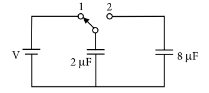Capacitance
Question

# A capacitor of $2\mu F$ is charged as shown in the diagram. When the switch $S$ is turned to position 2 , the percentage of its stored energy dissipated isDifficult
Solution

## initially, the charge stored in  $2\mu \mathrm{F}$ capacitor is$=\frac{\left({V}^{2}-{V}^{2}/5\right)×{10}^{-6}}{{V}^{2}×{10}^{-6}}×100%=80%$

Get Instant Solutions